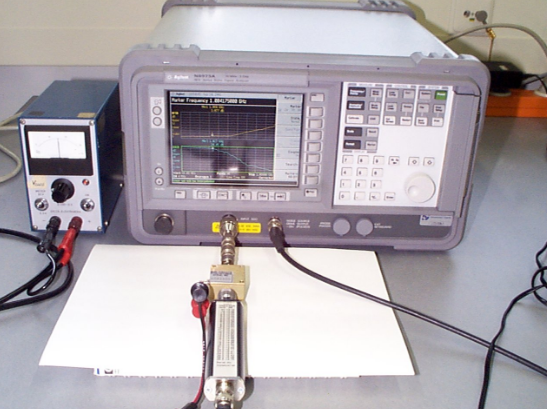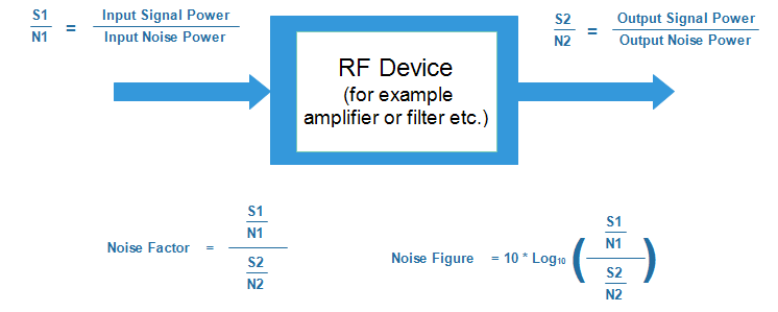# Noise Figure

Noise figure (NF) and noise factor (F) are measures of degradation of the signal-to-noise ratio (SNR), caused by components in a radio-frequency (RF) signal chain. It is a number by which the performance of an amplifier or a radio receiver can be specified, with lower values indicating better performance.

Not only is it widely used to assess the sensitivity performance or receivers, but it can be applied to complete receiving systems or to elements such as RF amplifiers. Thus it is possible to use the same notation to measure the noise performance of a whole receiver, or an RF amplifier.

Noise factor (F) is a measure of how the the signal to noise ratio is degraded by a device:

F = SNR(input) / SNR(output)

Where SNR is signal to noise ratio.

Noise figure (NF) is the noise factor, expressed in decibels:

NF (decibels) = 10 * log(F)